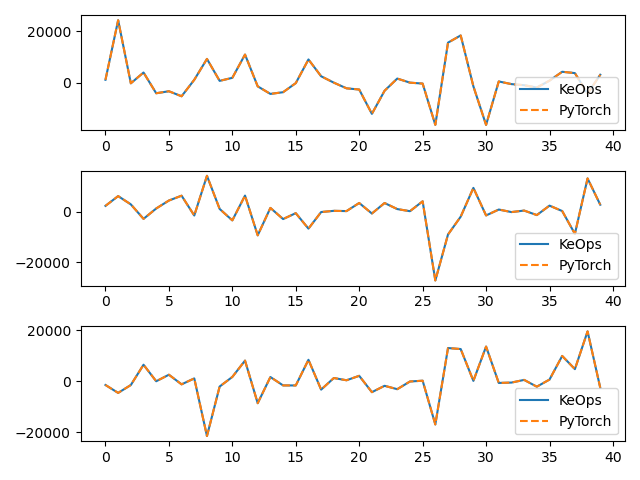Let’s write generic formulas using the KeOps syntax.

## Setup

First, the standard imports:

import torch
from pykeops.torch import Genred
import matplotlib.pyplot as plt

# Choose the storage place for our data : CPU (host) or GPU (device) memory.
device = torch.device("cuda" if torch.cuda.is_available() else "cpu")


Then, the definition of our dataset:

• $$p$$, a vector of size 2.

• $$x = (x_i)$$, an N-by-D array.

• $$y = (y_j)$$, an M-by-D array.

N = 1000
M = 2000
D = 3

# PyTorch tip: do not 'require_grad' of 'x' if you do not intend to
#              actually compute a gradient wrt. said variable 'x'.
#              Given this info, PyTorch (+ KeOps) is smart enough to
#              skip the computation of unneeded gradients.
x = torch.randn(N, D, requires_grad=False, device=device)
y = torch.randn(M, D, requires_grad=True, device=device)

# + some random gradient to backprop:
g = torch.randn(N, D, requires_grad=True, device=device)


## Computing an arbitrary formula

Thanks to the Elem operator, we can now compute $$(a_i)$$, an N-by-D array given by:

$a_i = \sum_{j=1}^M (\langle x_i,y_j \rangle^2) (p_0 x_i + p_1 y_j)$

where the two real parameters are stored in a 2-vector $$p=(p_0,p_1)$$.

# Keops implementation.
# Note that Square(...) is more efficient than Pow(...,2)
formula = "Square((X|Y)) * ((Elem(P, 0) * X) + (Elem(P, 1) * Y))"
variables = [
"P = Pm(2)",  # 1st argument,  a parameter, dim 2.
"X = Vi(3)",  # 2nd argument, indexed by i, dim D.
"Y = Vj(3)",
]  # 3rd argument, indexed by j, dim D.

my_routine = Genred(formula, variables, reduction_op="Sum", axis=1)
a_keops = my_routine(p, x, y)

# Vanilla PyTorch implementation
scals = (torch.mm(x, y.t())) ** 2  # Memory-intensive computation!
a_pytorch = p * scals.sum(1).view(-1, 1) * x + p * (torch.mm(scals, y))

# Plot the results next to each other:
for i in range(D):
plt.subplot(D, 1, i + 1)
plt.plot(a_keops.detach().cpu().numpy()[:40, i], "-", label="KeOps")
plt.plot(a_pytorch.detach().cpu().numpy()[:40, i], "--", label="PyTorch")
plt.legend(loc="lower right")
plt.tight_layout()
plt.show()Total running time of the script: ( 0 minutes 0.136 seconds)

Gallery generated by Sphinx-Gallery